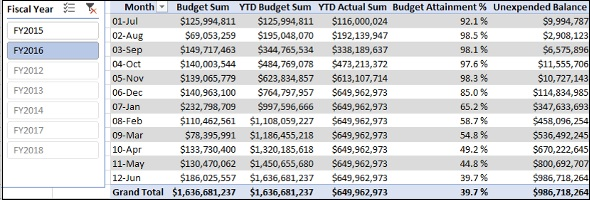# Budget Measures and Analysis

Budgeting involves estimating the cash flows of a company over a financial year. The financial position of the company, its goals, expected revenues, and expenses are taken into account in budgeting.

However, the market conditions may change during the financial year and the company may have to reset its goals. This requires analyzing the financial data with the budget estimated at the beginning of the financial year (Budget Sum) and the actual expended sum from the beginning of the financial year to date (YTD Actual Sum).

At any time during a financial year, you can calculate the following −

### Unexpended Balance

Unexpended Balance is the budget remaining after the actual expenses, i.e.

Unexpended Balance = YTD Budget Sum – YTD Actual Sum

### Budget Attainment %

Budget Attainment % is the percentage of the budget that you have spent to date, i.e.

Budget Attainment % = YTD Actual Sum/YTD Budget Sum

These calculations help those companies that use budgeting to make decisions.

### Creating Unexpended Balance Measure

You can create Unexpended Balance measure as follows −

Unexpended Balance:=CALCULATE( [YTD Budget Sum],ALL('Finance Data'[Date]) )-[YTD Actual Sum]

### Creating Budget Attainment Percentage Measure

You can create Budget Attainment Percentage measure as follows −

Budget Attainment %:=IF([YTD Budget Sum],[YTD Actual Sum]/CALCULATE([YTD Budget Sum],ALL('Finance Data'[Date])),BLANK())

## Analyzing Data with Budget Measures

Create a Power PivotTable as follows −

• Add Month from the Date table to Rows.

• Add the measures Budget Sum, YTD Budget Sum, YTD Actual Sum, Budget Attainment % and Unexpended Balance from Finance Data table to Values.

• Insert a Slicer on the Fiscal Year field.

• Select FY2016 in the Slicer.